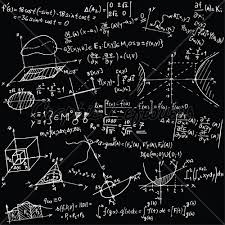# Summands 7380

Divide the number 4600 into two summands so that one summand is 700 larger than half of the other summand.

a =  2000
b =  2600

### Step-by-step explanation:

a+b=4600
a = 700 + b/2

a+b = 4600
2a-b = 1400

Pivot: Row 1 ↔ Row 2
2a-b = 1400
a+b = 4600

Row 2 - 1/2 · Row 1 → Row 2
2a-b = 1400
1.5b = 3900

b = 3900/1.5 = 2600
a = 1400+b/2 = 1400+2600/2 = 2000

a = 2000
b = 2600

Our linear equations calculator calculates it.Did you find an error or inaccuracy? Feel free to write us. Thank you!

Tips for related online calculators
Do you have a system of equations and looking for calculator system of linear equations?